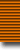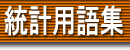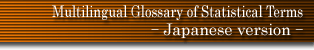### 用語検索

and or
検索単位 単語 フレーズ 完全一致 〜で始まる 〜を含む 〜で終わる 日本語 英語 ドイツ語 フランス語
 日本語 英語 ドイツ語 フランス語
 Gabriel's test Gabriel-Sen statistic gain function Galton ogive Galton-McAllister distribution Galton's individual difference problem Galton's rank order test Galton-Watson process gambler's ruin game theory gamma coefficients gamma distribution Gani's mean difference Gantt progress chart Gart's test Garwood distribution Gauss distribution Gauss elimination Gaussian distribution Gauss-Markov theorem Gauss-Poisson distribution Gauss-Seidel method Gauss-Winckler inequality Geary's contiguity ratio Geary's ratio Geary's theorem general factor general interdependent system general renewal process generalised Bayes' decision rule generalised binomial distribution generalised bivariate exponential distribution generalised classical linear estimators generalised contagious distribution generalised distance generalised distribution generalised gamma distributions generalised inverse generalised least squares estimator generalised linear model generalised maximum likelihood estimator generalised multinomial distribution generalised Normal distribution generalised polykays generalised power series distribution generalised right angular designs generalised sequential probability ratio test generalised stable law generalised STER distribution generalised T2 distribution generating function geometric distribution geometric mean geometric moving average geometric probability geometric range geostatistics Gibrat distribution Gini's hypothesis Gini's index of cograduation Gini's mean distance Gini's ratio girdle distribution Gittins index given period Glivenko-Cantelli lemma Glivenko's theorem glyph Gnedenko-Koroljuk distributions Gnedenko-Koroljuk theorem Gnedenko's theorem Gompertz curve Goodman-Kruskal G statistic Goodman-Kruskal measures of association Goodman-Kruskal tau goodness of fit Goutereau's constant Gower's similarity coefficient grade grade correlation graduation graduation curve Graeco-Latin square Gram-Charlier series type A Gram-Charlier series type B Gram-Charlier series type C Gramian Gramian matrix Gram's criterion graphical estimator Greenhouse-Geisser test Grenander's uncertainty principle grid grid sampling group group comparison group divisible design group divisible incomplete block design group divisible PBIB group divisible rotatable designs group factor group screening methods grouped Poisson distribution grouping lattice group-sequential design growth curve Grubbs' estimators Grubbs' rule g-statistics g-test Gumbel distribution Gumbel's inequalities Gupta's subset selection method Gupta's symmetry test Gurland's generalisation of Neyman's distribution Guttman scaling